﻿ Predictability of the Impact Strength of CaCO<SUB>3</SUB>-Epoxy Resin Composite Based on CaCO<SUB>3</SUB> Input Concentration and Sustained Stress at Impact

### Predictability of the Impact Strength of CaCO3-Epoxy Resin Composite Based on CaCO3<...

C. I. Nwoye, M. C. Obelle, S. O. Nwakpa, C. W. Onyia, I. Obuekwe, N. E. Idenyi

## Predictability of the Impact Strength of CaCO3-Epoxy Resin Composite Based on CaCO3 Input Concentration and Sustained Stress at Impact

C. I. Nwoye1,, M. C. Obelle2, S. O. Nwakpa1, C. W. Onyia3, I. Obuekwe4, N. E. Idenyi5

1Department of Metallurgical and Materials Engineering, Nnamdi Azikiwe University Awka, Anambra State, Nigeria

2Department of Polymer and Textile Engineering, Nnamdi Azikiwe University Awka, Anambra State, Nigeria

3Department of Metallurgical and Materials Engineering, Enugu State University of Science & Technology, Enugu State, Nigeria

4Scientific Equipment Development Institute Enugu, Nigeria

5Department of Industrial Physics, Ebonyi State University, Abakiliki, Nigeria

### Abstract

This paper presents the predictability of the impact strength of calcium carbonate (CaCO3) reinforced epoxy resin composite based on CaCO3 Input Concentration (CIC) and impact load referred to as Sustained Stress At Impact (SSAI) which is the impact load. Results from experiment, derived and regression model prediction show that the impact strength of CaCO3-epoxy resin composite increase with decrease in CIC and increase in SSAI. A two-factorial model was derived, validated and used for the predictive analysis. The derived model showed that the impact strength of CaCO3-epoxy resin composite is a linear function of CIC and SSAI. The validity of the derived model expressed as: ξ = 0.2223 ₰ - 0.0532 ϑ + 9.3519 was rooted on the model core expression ξ – 9.3519 = 0.2223₰ - 0.0532 ϑ where both sides of the expression are correspondingly approximately equal. Results from evaluations indicated that the standard error incurred in predicting CaCO3-epoxy resin composite impact strength for each value of the CIC & SSAI considered, as obtained from experiment, derived model and regression model were 0.3807, 0.3698 and 2.9277 x 10-5 & 0.8081, 0.3909 and 0.7808 % respectively. The composite impact strength per unit CIC as obtained from experimental, derived model and regression model predicted results are 0.1175, 0.1088 and 0.1063 J/m %-1, respectively and the correlations with CIC & SSAI were all > 0.9. Maximum deviation of model-predicted CaCO3-epoxy resin composite impact strength from the experimental results is 7.45%. These invariably translated into over 92% operational confidence for the derived model as well as over 0.92 reliability response coefficients of CaCO3-epoxy resin composite impact strength to CIC and SSAI.

• C. I. Nwoye, M. C. Obelle, S. O. Nwakpa, C. W. Onyia, I. Obuekwe, N. E. Idenyi. Predictability of the Impact Strength of CaCO3-Epoxy Resin Composite Based on CaCO3 Input Concentration and Sustained Stress at Impact. International Journal of Materials Lifetime. Vol. 2, No. 1, 2015, pp 6-12. http://pubs.sciepub.com/ijml/2/1/2
• Nwoye, C. I., et al. "Predictability of the Impact Strength of CaCO3-Epoxy Resin Composite Based on CaCO3 Input Concentration and Sustained Stress at Impact." International Journal of Materials Lifetime 2.1 (2015): 6-12.
• Nwoye, C. I. , Obelle, M. C. , Nwakpa, S. O. , Onyia, C. W. , Obuekwe, I. , & Idenyi, N. E. (2015). Predictability of the Impact Strength of CaCO3-Epoxy Resin Composite Based on CaCO3 Input Concentration and Sustained Stress at Impact. International Journal of Materials Lifetime, 2(1), 6-12.
• Nwoye, C. I., M. C. Obelle, S. O. Nwakpa, C. W. Onyia, I. Obuekwe, and N. E. Idenyi. "Predictability of the Impact Strength of CaCO3-Epoxy Resin Composite Based on CaCO3 Input Concentration and Sustained Stress at Impact." International Journal of Materials Lifetime 2, no. 1 (2015): 6-12.

 Import into BibTeX Import into EndNote Import into RefMan Import into RefWorks

1234
Prev Next

### 1. Introduction

The wide scope usability of epoxy resin for industrial and domestic purposes has necessitated various researches aimed at improving its quality for a better product performance and durability.

Epoxy has a wide range of industrial applications, including metal coatings, use in electronic and electrical components, high tension electric insulators, fibre-reinforced plastic materials, and structural adhesive. Epoxy resin is employed to bind gutta percha in some root canal procedures . Epoxy resins are also used for decorative flooring applications such as terrazzo flooring, chip flooring, and colored aggregate flooring. Epoxy flooring has been proven to be an environmentally friendly alternate to other types of flooring, reducing the facility’s impact on the environment through less water consumption and less pesticide needed .

Epoxy resins, also known as polyepoxide are a class of reactive prepolymers and polymer which contain epoxide groups. Epoxy resins may be reacted (cross linked) either with themselves through catalytic homopolymerization, or with a wide range of co-reactants including polyfunctional amines, acids (and acid anhydrides), phenols, alcohols, and thiols. Research  shows that there two types of aliphatic epoxy resins: glycidyl epoxy resins and cycloaliphatic epoxide. The cycloaliphatic epoxides are formed by the reaction of cyclo-olefins with a peracid, such as perac1etic acid . This class also displays low viscosity at room temperature, but offers significantly higher temperature resistance and correspondingly better electrical properties at high temperatures to cured resins than the glycidyl aliphatic epoxy resins.

The applicability of a polymeric material in service depends on a good understanding of the degree of resistance of this material to impact loading. As with metals, polymers may exhibit ductile or brittle fracture under impact loading conditions, depending on the temperature, specimen size, strain rate, and mode of loading . The report shows that both semicrystalline and amorphous polymers are brittle at low temperatures, and both have relatively low impact strengths. However, they experience a ductile-to-brittle transition over a relatively narrow temperature range.

It has been shown  that the matrix often determines the maximum service temperature, since it normally softens, melts, or degrades at a much lower temperature than the fiber reinforcement.

The most widely utilized and least expensive polymer resins are the polyesters and vinyl esters . These matrix materials are used primarily for glass fiber-reinforced composites. A large number of resin formulations provide a wide range of properties for these polymers. The epoxies are more expensive and, in addition to commercial applications, are also utilized extensively in PMCs for aerospace applications because they have better mechanical properties and resistance to moisture than the polyesters and vinyl resins .

For high-temperature applications, polyetherimide resins are employed . The scientist also reported that high-temperature thermoplastic resins offer the potential for usage in future aerospace applications. Such materials were reported to include polyetheretherketone (PEEK), polyphenylene sulfide (PPS), and polyetherimide (PEI).

Polymer-matrix composites (PMCs) consist of a polymer resin  as the matrix, with fibers as the reinforcement medium. The report revealed that the materials are used in the greatest diversity of composite applications, as well as in the largest quantities, due to their room-temperature properties, ease of fabrication, and cost.

The relationship between reinforcing fillers and compressive strength has been evaluated  based on the use of short glass fibres. Similar research  posited that polymers can only improve their young’s modulus if filled by right inorganic particles. However, report  has shown that fillers also lead to reduction of the fracture strain and even to embrittlement of polymers.

Researchers  have evaluated the interaction between the polymer matrix, calcium carbonate and silica filler on the basis of acid-base interaction. Successful study  on the acid-base interaction in polymer matrix/filler systems has shown that the interaction significantly depends on the pH, the size, composition, nature, distribution, and compatibility of the components in the system.

Scientists  have studied the effect of different percentage compositions of silica and CaCO3 fillers on the tensile strength of epoxy resin. However, the percentage substitution of silica filler with CaCO3 was limited to a maximum of 3%. Other researchers  studied the effect of aggregate (SiO2) size on the compressive strength of PMMA synthetic marble containing fixed amount of CaCO3. In all these studies, the effect of varying amounts of CaCO3 and SiO2 fillers on a fixed ratio of epoxy resin to filler system (CaCO3/SiO2) was not investigated. On the other hand, past researchers have not addressed the need to have a model or models that can predict the toughness, impact strength, tensile strength or compressive strength of CaCO3-epoxy resin composite based on the various amount of CaCO3 added to epoxy resin.

Polymers may experience fatigue failure under conditions of cyclic loading. As with metals, fatigue occurs at stress levels that are low relative to the yield strength . Fatigue testing in polymers has not been nearly as extensive as with metals; however, fatigue data are plotted in the same manner for both types of material, and the resulting curves have the same general shape.

Some polymers have a fatigue limit (a stress level at which the stress at failure becomes independent of the number of cycles) ; others do not appear to have such a limit. As would be expected, fatigue strengths and fatigue limits for polymeric materials are much lower than for metals. The fatigue behavior of polymers is much more sensitive to loading frequency than for metals. Cycling loading of polymers at high frequencies and/or relatively large stresses can cause localized heating; consequently, failure may be due to a softening of the material rather than as a result of typical fatigue processes .

The magnitude of the tensile modulus does not seem to be directly influenced by molecular weight. On the other hand, for many polymers it has been observed that tensile strength increases with increasing molecular weight . Mathematically, TS is a function of the number-average molecular weight according to :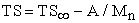(1)

Where TS is the tensile strength at infinite molecular weight and A is a constant. The behavior described by this equation is explained by increased chain entanglements with rising Mn

The present work aims to predict the impact strength of CaCO3-epoxy resin composite based on the values of the CIC and SSAI (impact load). An empirical model will be derived, validated and corresponding values of input parameters: CIC and SSAI substituted into, to evaluate the impact strength which shall thereafter be compared with the experimentally determined impact strength. This research idea was culled from the fact that when a material experiences impact loading, it is stressed and strain is created prior to failure. The model that will be derived is expected give a direct prediction of the impact strength of the composite (providing the values of CIC and SSAI are known). Based on the foregoing, this model ensures calculation of the impact strength of the composite when CIC and SSAI are known instead of carrying out the Izod test each time to evaluate the impact strength.

### 2. Materials and Method

Materials used for the production of CaCO3-epoxy resin composite includes epoxy resin (which is of German origin with relative density of 1.2), polyamine catalyst, (dark in color and packaged with the epoxy resin), calcium carbonate (CaCO3) which is white in colour with relative density of about 1.4, particle size of between 0.5-0.06mm and manufactured by Kavitex Nigeria Limited.

Production of Caco3-Epoxy Resin Composite

The filler powders were dispersed in the liquid epoxy resin components by stirring gently and continuously. This was followed by the addition of the catalyst or curing agent. Finally the mixtures were poured into series of moulds of the same shape and compacted by vibration at room temperature. Epoxy resin pre-polymer was catalysed with a tertiary polyamine to give a thermoset; epoxy plastic. The curing or cross linking process was observed by the gradual build-up of the viscosity and increase in temperature of the mixture.

Impact strength Testing

The moulds were made according to the shape designate of ASTM D256-81  for impact test samples. ASTM D256  testing procedure was adopted for the impact tests. The Izod test method A was used.

Standard specimen size (in accordance with ASTM D256-81) was used for the impact test. The specimen was dimensioned; 64 x 12.7 x 3.2 mm. The depth under the notch of the specimen was 10.2 mm. ASTM  impact energy is expressed in J/m. Impact strength was evaluated by dividing impact energy in J by the thickness of the specimen (3.2 mm).

### 3. Results and Discussions

Table 1 shows that the impact strength of CaCO3-epoxy resin composite increases with increase in SSAI and decrease in CIC.

#### Table 1. Variation of CaCO3-epoxy resin composite impact strength with CIC and SSAIDownload asVeiw tableTables index
3.1. Model Formulation

Computational analysis (using C-NIKBRAN: ) of results in Table 1 indicates that(2)

Substituting the values of K, N and S into equation (2) reduces it to;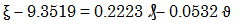(3)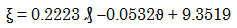(4)

Where

K = 9.3519; N = 0.2223 and S = 0.0532 are equalizing constants (Determined using C-NIKBRAN )

(ξ) = Impact strength of CaCO3-epoxy resin composite (J/m)

(₰) = SSAI = Impact load (MPa)

(ϑ) = CIC (%)

3.1.1. Boundary and Initial Conditions

Considered ranges of CaCO3-epoxy resin composite impact strength, CIC and SSAI are 8.51 – 14.20 J/m, 10 - 60% and 11.80 - 22.5 (MPa) respectively.

3.1.2. Model validation

The validity of the derived model was rooted in equation (3) where both sides of the equation are correspondingly approximately almost equal. Furthermore, equation (3) agrees with Table 2 following the values of ξ – 9.3519 and 0.2223 ₰ - 0.0532 ϑ evaluated from Table 1.

#### Table 2. Variation of ξ – 9.3519 with 0.2223 ₰ - 0.0532 ϑDownload asVeiw tableTables index

Furthermore, the derived model was validated by comparing the model-predicted impact strength of CaCO3-epoxy resin composite and that obtained from the experiment. This was done using the 4th Degree Model Validity Test Techniques (4thDMVTT); statistical graphical, computational and deviational analysis.Download asVeiw figureFigures index
Figure 1. Variation of CaCO3-epoxy resin composite impact strength with CIC as obtained from experimentDownload asVeiw figureFigures index
Figure 2. Variation of CaCO3-epoxy resin composite impact strength with CIC as predicted by derived modelDownload asVeiw figureFigures index
Figure 3. Variation of CaCO3-epoxy resin composite impact strength with SSAI as obtained from experimentDownload asVeiw figureFigures index
Figure 4. Variation of CaCO3-epoxy composite impact strength with SSAI as predicted by derived model
3.2. Statistical Analysis
3.2.1. Standard Error (STEYX)

The standard errors incurred in predicting the impact strength of CaCO3-epoxy resin composite for each value of the CIC & SSAI considered as obtained from experiment and derived model were 0.3807 and 0.3698 & 0.8081 and 0.3909 % respectively. The standard error was evaluated using Microsoft Excel version 2003.

3.2.2. Correlation (CORREL)

The correlation coefficient between impact strength of CaCO3-epoxy resin composite and CIC & SSAI were evaluated (using Microsoft Excel Version 2003) from results of the experiment and derived model. These evaluations were based on the coefficients of determination R2 shown in Figure 1- Figure 4.(5)

The evaluated correlations are shown in Table 3 and Table 4. These evaluated results indicate that the derived model predictions are significantly reliable and hence valid considering its proximate agreement with results from actual experiment.

#### Table 3. Comparison of the correlations evaluated from derived model predicted and experimental results based on CICDownload asVeiw tableTables index

#### Table 4. Comparison of the correlations evaluated from derived model predicted and experimental results based on SSAIDownload asVeiw tableTables index
3.3. Graphical Analysis

Comparative graphical analysis of Figure 5 and Figure 6 show very close alignment of the curves from the experimental (ExD) and model-predicted (MoD) impact strength of CaCO3-epoxy resin composite.Download asVeiw figureFigures index
Figure 5. Comparison of CaCO3-epoxy resin composite impact strengths (relative to CIC) as obtained from experiment  and derived modelDownload asVeiw figureFigures index
Figure 6. Comparison of CaCO3-epoxy resin composite impact strengths (relative to SSAI) as obtained from experiment and derived model

It is strongly believed that the degree of alignment of these curves is indicative of the proximate agreement between both experimental and model-predicted values of the CaCO3-epoxy resin composite impact strength.

3.3.1. Comparison of Derived Model with Standard Model

The validity of the derived model was further verified through application of the Least Square Method (LSM) in predicting the trend of the experimental results.Download asVeiw figureFigures index
Figure 7. 3-D Comparison of CaCO3-epoxy resin composite impact strengths (relative to CIC) as obtained from ExD, MoD and ReG

Comparative analysis of Figure 7 and Figure 8 shows very close alignment of curves and areas covered by impact strength of CaCO3-epoxy resin composite, which precisely translated into significantly similar trend of data point’s distribution for experimental (ExD), derived model (MoD) and regression model-predicted (ReG) results of impact strengths of CaCO3-epoxy resin composite.

Also, the calculated correlations (from Figure 7 and Figure 8) between impact strength of CaCO3-epoxy resin composite and CIC & SSAI for results obtained from regression model gave 1.0000 & 0.9363 respectively. These values are in proximate agreement with both experimental and derived model-predicted results. Comparative analysis of the correlations between impact strength of CaCO3-epoxy resin composite and CIC & SSAI as obtained from experiment, derived model and regression model indicated that they were all > 0.9.Download asVeiw figureFigures index
Figure 8. 3-D Comparison of CaCO3-epoxy resin composite impact strengths (relative to SSAI) as obtained from ExD, MoD and ReG

The standard errors incurred in predicting the impact strength of CaCO3-epoxy resin composite for each value of the CIC & applied load considered as obtained from regression model were 2.9277 x10-5 and 0.7808% respectively.

3.4. Computational Analysis

Computational analysis of the experimental and model-predicted impact strength of CaCO3-epoxy resin composite was carried out to ascertain the degree of validity of the derived model. This was done by comparing the impact strength of CaCO3-epoxy resin composite per unit CIC obtained from evaluation of experimental and model-predicted results.

3.4.1. Impact Strength of CaCO3-epoxy Resin Composite Per Unit CIC

The impact strength of CaCO3-epoxy resin composite per unit CIC was calculated from the expression;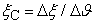(6)

Equation (6) is detailed as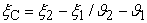(7)

Where

Δξ = Change in impact strength at two values of CIC ϑ 2, ϑ 1.

Considering the points (50, 9.5) & (10, 14.2), (50, 9.4707) & (10, 13.8217) and (50,9.5657) & (10, 13.8171) as shown in Fig. 8, then designating them as (ζ1, ϑ1) & (ζ2, ϑ2) for experimental, derived model and regression model predicted results respectively, and also substituting them into equation (7), gives the slopes: - 0.1175, - 0.1088 and - 0.1063 J/m %-1 as their respective CaCO3-epoxy resin composite impact strength per unit CIC.

It is very pertinent to state that the actual CaCO3-epoxy resin composite impact strength per unit CIC (as obtained from experiment and derived model) was just the magnitude of the signed value. The associated sign preceding these values as evaluated signifies that the associated slope tilted to negative plane. Based on the foregoing, the impact strength of CaCO3-epoxy resin composite per unit CIC as obtained from experimental, derived model and regression model predicted results are 0.1175, 0.1088 and 0.1063 J/m %-1, respectively.

3.5. Deviational Analysis

Critical analysis of the impact strength of CaCO3-epoxy resin composite obtained from experiment and derived model show low deviations on the part of the model-predicted values relative to values obtained from the experiment. This was attributed to the fact that the surface properties of the CaCO3 and epoxy resin and also the physico-chemical interactions between the carbonate and epoxy resin plastic which played vital roles during processing were not considered during the model formulation. This necessitated the introduction of correction factor, to bring the model-predicted impact strength of CaCO3-epoxy resin composite to those of the corresponding experimental values.

The deviation Dv, of model-predicted impact strength of CaCO3-epoxy resin composite (from the corresponding experimental result) is given by(8)

Where

ξExD and ξMoD are impact strength of CaCO3-epoxy resin composite obtained from experiment and derived model respectively.

Deviational analysis of Figure 9 and Figure 10 indicate that the precise maximum deviation of model-predicted CaCO3-epoxy resin composite impact strength from the experimental results is 7.45%. This invariably translated into over 92% operational confidence for the derived model as well as over 0.92 reliability response coefficients of CaCO3-epoxy resin composite impact strength to CaCO3 addition and applied load.Download asVeiw figureFigures index
Figure 9. Variation of model-predicted impact strength of CaCO3-epoxy resin composite (relative to CIC) with associated deviation from experiment

Consideration of equation (8) and critical analysis of Figure 9 and Figure 10 show that the least and highest magnitudes of deviation of the model-predicted CaCO3-epoxy resin composite impact strength (from the corresponding experimental values) are – 0.31 and – 7.45%. Figure 9 and Figure 10 indicate that these deviations correspond to CaCO3-epoxy resin composite impact strength: 9.4707 and 10.1805 J/m, CIC: 50 and 40 % as well as SSAI: 12.5 and 13.3 MPa respectively.Download asVeiw figureFigures index
Figure 10. Variation of model-predicted impact strength of CaCO3-epoxy resin composite (relative to applied load) with associated deviation from experiment.

Correction factor, Cf to the model-predicted results is given by(9)

Critical analysis of Figure 9, Figure 10 and Table 5 indicates that the evaluated correction factors are negative of the deviation as shown in equations (8) and (9).

#### Table 5. Variation of correction factor (to model-predicted impact strength of CaCO3-epoxy resin composite) with CIC and SSAIDownload asVeiw tableTables index

Table 5 shows that the least and highest correction factor (to the model-predicted impact strength of CaCO3-epoxy resin composite) are + 0.31 and + 7.45%. Since correction factor is the negative of deviation as shown in equations (8) and (9), Table 5, Figure 9 and Figure 10 indicate that these highlighted correction factors corresponds to CaCO3-epoxy resin composite impact strength: 9.4707 and 10.1805 J/m, CIC: 50 and 40 % as well as SSAI: 12.5 and 13.3 MPa respectively.

The correction factor took care of the negligence of operational contributions of the surface properties of the CaCO3 and epoxy resin, and also the physico-chemical interactions between the carbonate and epoxy resin plastic which actually played vital role during processing. The model predicted results deviated from those of the experiment because these contributions were not considered during the model formulation. Introduction of the corresponding values of Cf from equation (9) into the model gives exactly the corresponding experimental values of CaCO3-epoxy resin composite impact strength.

It is very pertinent to state that the deviation of model predicted results from that of the experiment is just the magnitude of the value. The associated sign preceding the value signifies that the deviation is a deficit (negative sign) or surplus (positive sign).

### 4. Conclusion

The impact strength of calcium carbonate (CaCO3) reinforced epoxy resin composite was predicted (with the aid of an empirical model) based on known values of the CIC and SSAI. The impact strength of CaCO3-epoxy resin composite increases with decrease in CIC and increase in SSAI. The derived model (validated and used) showed that the impact strength of CaCO3-epoxy resin composite is a linear function of CIC and SSAI. The validity of the derived model was rooted in the model core expression ξ – 9.3519 = 0.2223 ₰ - 0.0532 ϑ where both sides of the expression are correspondingly approximately equal. The standard error incurred in predicting CaCO3-epoxy resin composite impact strength for each value of the CIC & SSAI considered, as obtained from experiment, derived model and regression model were 0.3807, 0.3698 and 2.9277 x 10-5 & 0.8081, 0.3909 and 0.7808 % respectively. Evaluated results indicate that CaCO3-epoxy resin composite impact strength per unit CIC as obtained from experimental, derived and regression model predicted results are 0.1175, 0.1088 and 0.1063 J/m %-1 respectively. The correlations between impact strength of CaCO3-epoxy resin composite and CIC & SSAI as obtained from experiment, derived and regression model indicated that they were all > 0.9. Maximum deviation of model-predicted CaCO3-epoxy resin composite impact strength from the experimental results is 7.45%. These invariably translated into over 92% operational confidence for the derived model as well as over 0.92 reliability response coefficients of CaCO3-epoxy resin composite impact strength to CIC and SSAI.

### References

  Marciano, M. A., Ordinola-Zapata, R., Cunhu, T. V. R. N., , M. A.. H., Cavenago, B. C., Garcia, R. B., Bramante, C. M., Bernardineli, N., Moraes, G. (2011). Analysis of Four Gutta-Percha Techniques Used to Fill Mesial Root Canals of Mandibular Molars. International Endodontic Journal 44(4): 321-329.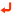In article View Article  PubMed  Environmentally Friendly Epoxy Floors. Everlastepoxy.com. 2014.In article  Epoxy Resins Chemistry and Technology, 1988 Marcel Dekker, Chapter 2: Synthesis and Characterization of Epoxides, by Yoshio Tanaka, pp. 54-63.In article  Recent Developments in Epoxy Resins, by L. Hammerton, RAPRA Review Reports, 1997, ed by Rebecca Dolbey pp. 8In article  Callister Jnr, W. D. (2007). Materials Science and Engineering, 7th Edition, John Wiley & Sons, USA, pp. 539-563.In article  Agarwal, B. D. and Broutman, L. J. (1990). Analysis and Performance of Fiber Composites, 2nd edition, Wiley, New York, pp 34-52.In article PubMed  Allen, K. W. (1987), (ed), Adhesion, vol. 12, Elsevier Applied Science, London, pp. 33-38.In article  Bazhenor S. J. (1995), Polym. Eng. & Sci, 35(10):813.In article View Article  Fridrich, K. and Karson, U.A. (1983). Fibre Science Technol, 19:37.In article View Article  Fowkes, F.M. J. (1987). Adhesion Sci. and Technol 2(1):7In article View Article  Usmani, A. M., (1982). Polym – Plastic, Technol. Eng; 19(2):180-181.In article View Article  Maisel, J. W. and Waso, S.K., (1982). J. Polym. – Plastics (technology and Engineering), Ed. Louis Naturman, 19 (2):227-242.In article  Enyiegbulam, M. E. (1992). Int. J. Adhesion and Adhesives, 12(2):118-119.In article View Article  Ashbee, K. H. (1993). Fundamental Principles of Fiber Reinforced Composites, 2nd edition, Technomic Publishing `Company, Lancaster, PA. pp. 36.In article PubMed  American Standard Test Method (ASTM D 256) For Measuring Impact Strength.In article  Enyiegbulam, M. E. and Okoro, U. D. (2008). Acid-Base Interactions in Epoxy Resin Marble Synthesis, Int. Res. J. Eng. Sc., Tech., 5(1): 16-28.In article  Nwoye, C. I. (2008). Data Analytical Memory; C-NIKBRAN.In article PubMed